Get inspired by the success stories of our students in IIT JAM MS, ISI  MStat, CMI MSc DS.  Learn More

# Neyman Welcomes You | ISI MStat 2018 PSB Problem 8This is a problem from ISI MStat Examination,2018. It involves the construction of the most powerful test of size alpha using Neyman Pearson Lemma. The aim is to find its critical region in terms of quantiles of a standard distribution.

## Problem

Let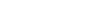be an i.i.d sample from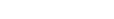, with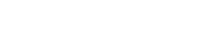and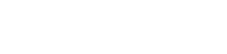Based on the above sample,obtain the most powerful test for testing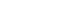against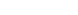, at level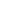, with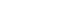.Find the critical region in terms of quantiles of a standard distribution.

## Prerequisites

1. The Fundamental Neyman Pearson Lemma

2. Useful Transformations of Random Variables

3. Properties of standard probability distributions (e.g. Normal,Chi-squared etc)

All these topics are included in the regular coursework of undergraduate statistics students. If not, one may refer standard texts like Casella Berger.

## Solution

As,is a random sample, they are independent by definition.
So, their joint pdf when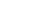is given by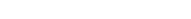, where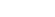denotes the indicator function over the interval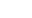.

Similarly, the joint pdf when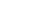is given by: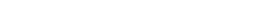According to the Fundamental Neyman Pearson Lemma}, the most powerful sizetest for testing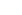vs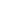is given by the test function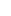as follows: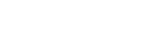where k is such that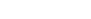.

So, our test criterion is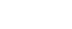Plugging in the pdfs, we get the criterion as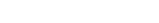(say)

Our aim now is to find the value of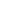from the given sizecriterion,
Thus,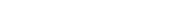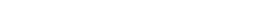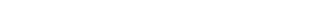Now, we state a result: If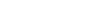,then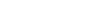distribution (Prove it yourself!)

As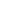's are independent, due to reproductive property of chi-squared distribution,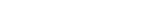Hence , we simply need that value ofsuch that the quantity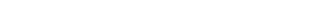The obvious choice is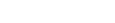, where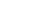is the upperpoint of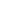distribution.

So, we haveimplies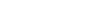So, our critical region for this test is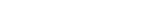## Food For Thought

In this problem , look at the supports of the two distributions under the null and alternative hypotheses.
See that both the supports are the same and hence the quantity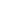is defined everywhere.
But suppose for a problem the two supports are not the same and they are not disjoint then try constructing a most powerful test using the Neyman Pearson Lemma.
For Example:
Let the family of distributions be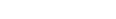Find the most powerful test for testingagainstNote that the supports under null and alternative hypotheses are not the same in this case.
Give it a try!

This is a problem from ISI MStat Examination,2018. It involves the construction of the most powerful test of size alpha using Neyman Pearson Lemma. The aim is to find its critical region in terms of quantiles of a standard distribution.

## Problem

Letbe an i.i.d sample from, withandBased on the above sample,obtain the most powerful test for testingagainst, at level, with.Find the critical region in terms of quantiles of a standard distribution.

## Prerequisites

1. The Fundamental Neyman Pearson Lemma

2. Useful Transformations of Random Variables

3. Properties of standard probability distributions (e.g. Normal,Chi-squared etc)

All these topics are included in the regular coursework of undergraduate statistics students. If not, one may refer standard texts like Casella Berger.

## Solution

As,is a random sample, they are independent by definition.
So, their joint pdf whenis given by, wheredenotes the indicator function over the interval.

Similarly, the joint pdf whenis given by:According to the Fundamental Neyman Pearson Lemma}, the most powerful sizetest for testingvsis given by the test functionas follows:where k is such that.

So, our test criterion isPlugging in the pdfs, we get the criterion as(say)

Our aim now is to find the value offrom the given sizecriterion,
Thus,Now, we state a result: If,thendistribution (Prove it yourself!)

As's are independent, due to reproductive property of chi-squared distribution,Hence , we simply need that value ofsuch that the quantityThe obvious choice is, whereis the upperpoint ofdistribution.

So, we haveimpliesSo, our critical region for this test is## Food For Thought

In this problem , look at the supports of the two distributions under the null and alternative hypotheses.
See that both the supports are the same and hence the quantityis defined everywhere.
But suppose for a problem the two supports are not the same and they are not disjoint then try constructing a most powerful test using the Neyman Pearson Lemma.
For Example:
Let the family of distributions beFind the most powerful test for testingagainstNote that the supports under null and alternative hypotheses are not the same in this case.
Give it a try!

This site uses Akismet to reduce spam. Learn how your comment data is processed.

### Knowledge Partner